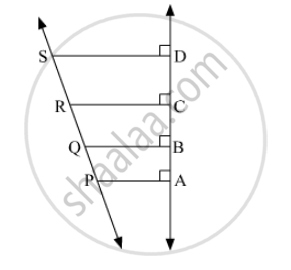# In the given figure, seg PA, seg QB, seg RC and seg SD are perpendicular to line AD. AB = 60, BC = 70, CD = 80, PS = 280 then Find PQ, QR and RS. - Geometry

In the given figure, seg PA, seg QB, seg RC and seg SD are perpendicular to line AD. AB = 60, BC = 70, CD = 80, PS = 280 then Find PQ, QR and RS.#### Solution

Given:
AB = 60,
BC = 70,
CD = 80,
PS = 280
Now, AD = AB + BC + CD
= 60 + 70 + 80
= 210
By intercept theorem, we have

$\frac{PQ}{AB} = \frac{QR}{BC} = \frac{RS}{CD} = \frac{PS}{AD}$
$\Rightarrow \frac{PQ}{60} = \frac{QR}{70} = \frac{RS}{80} = \frac{280}{210}$
$\Rightarrow \frac{PQ}{60} = \frac{QR}{70} = \frac{RS}{80} = \frac{4}{3}$

$\therefore PQ = \frac{4}{3} \times 60 = 80$
$QR = \frac{4}{3} \times 70 = \frac{280}{3}$
$RS = \frac{4}{3} \times 80 = \frac{320}{3}$

Concept: Similarity of Triangles
Is there an error in this question or solution?